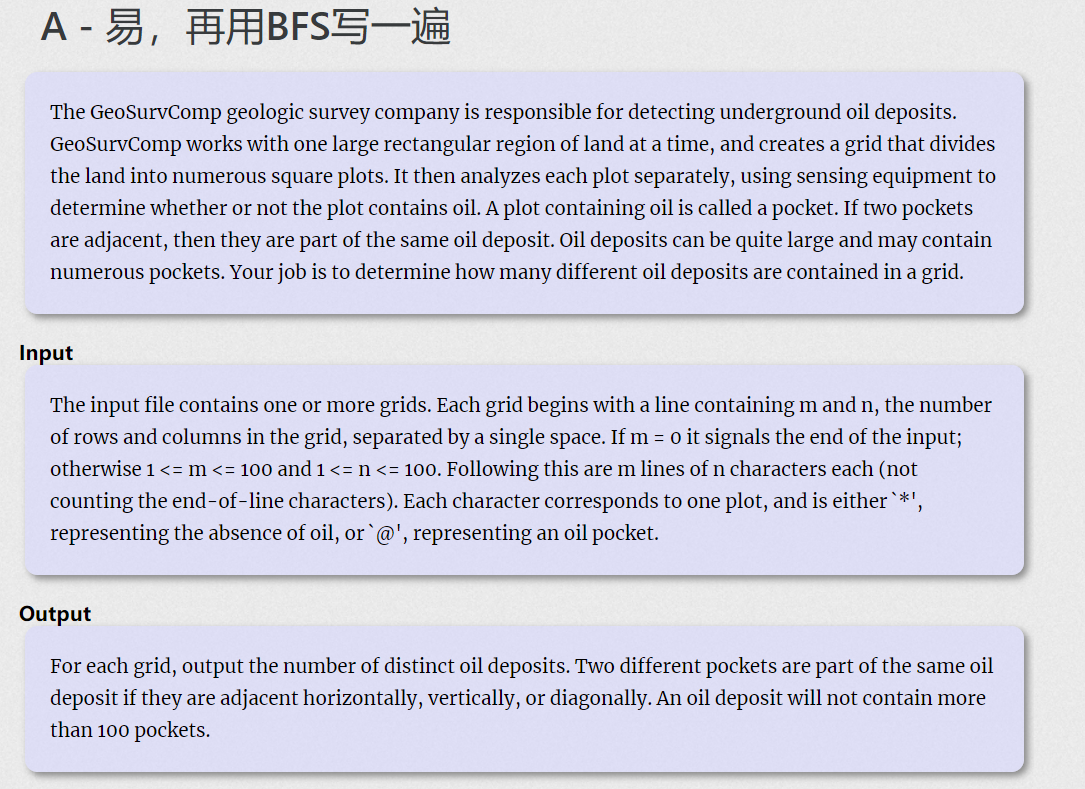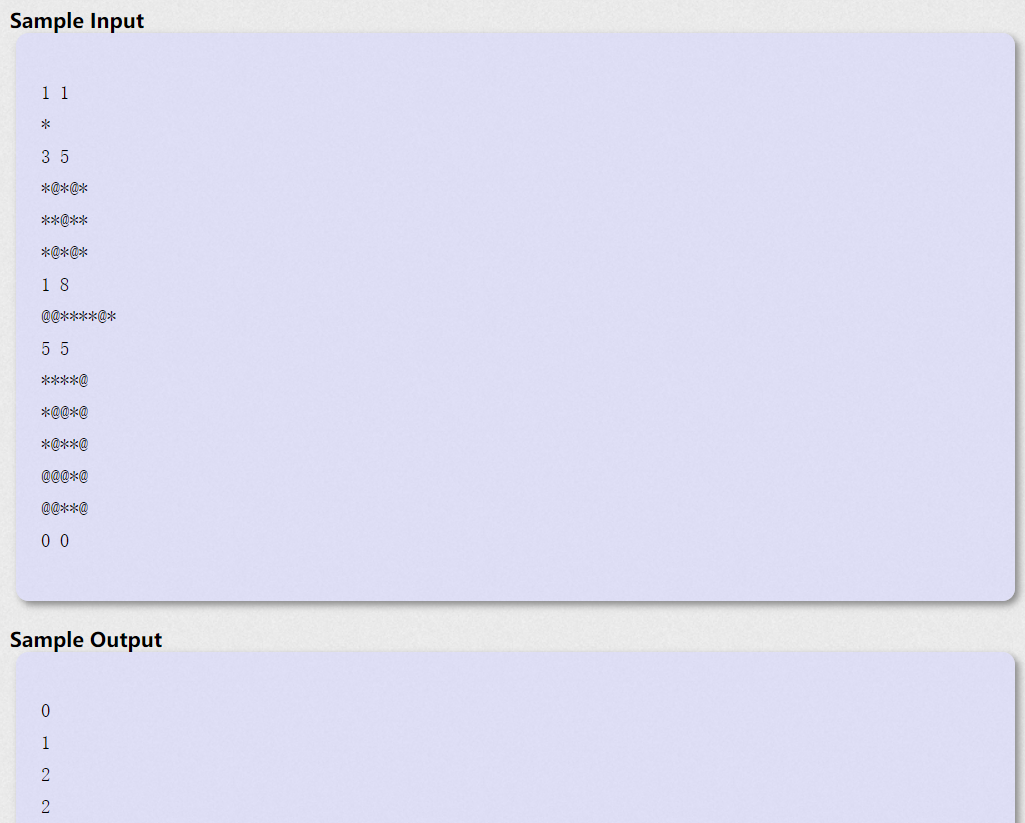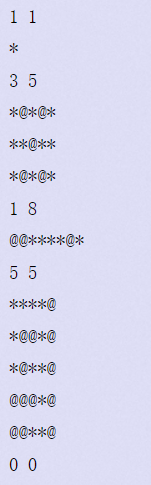• 时间:

# 题目链接：

https://vjudge.net/contest/345248#problem/A

# 题目# 翻译：

GeoSurvComp地质调查公司负责探测地下石油矿床GeoSurvComp一次处理一个大的矩形区域，并创建一个网格，将土地划分为许多正方形地块。然后，它分别分析每个图，使用传感设备来确定图中是否含有石油。一块含有石油的地皮叫做口袋。如果两个凹陷相邻，则它们是同一个油藏的一部分。石油储量可能相当大，并可能包含许多口袋。你的工作是确定一个网格中有多少不同的石油储量。0
1
2
2

# 参考代码：

``````#include<stdio.h>
#include<string.h>
#include<stdlib.h>
#include<math.h>
#include<algorithm>
using namespace std;
char a;//用来存储矩形区域的东西
int m=0,n=0,ans;//m代表行，n代表列，ans用于计数
int mark= {0};//标记被使用过的点的一个数组
int dx[]= {-1,-1,-1,0,0,1,1,1};
int dy[]= {-1,0,1,-1,1,-1,0,1};//这两个数组可以用来代表八个方向的遍历
void dfs(int x,int y,int z)
{
int xx,yy;
if(x<0||x>=m||y<0||y>=n)//如果越界就直接跳出这一个函数，跳出循环的条件
{
return ;
}
if(mark[x][y]>0||a[x][y]!='@')//被使用过的点或者没有油袋的点也直接跳过
{
return ;
}
mark[x][y]=1;//标记这个使用过的点
for(int i=0; i<=7; i++)
{
xx=x+dx[i];
yy=y+dy[i];
dfs(xx,yy,z);//对八个方向的遍历，每个方向都进行一次dfs函数，就可以首先把每个方向是否存在油袋，如果存在油袋，又继续判断那个油袋的八个方向是否存在油袋。这个就实现了不断判断的效果，直到在八个方向没有遇到油袋或者越界这个循环就会结束，这个油藏也结束了。
}
}

int main()
{
while(scanf("%d%d",&m,&n)&&(m+n)!=0)//多组输入，如果n，m为0则停止多组输入
{
ans=0;//每组样例都要重新把油藏数目清0.
memset(mark,0,sizeof(mark));//每组样例也要把标记的数组全部清0，重新标记
for(int i=0; i<m; i++)
{
scanf("%s",a[i]);//输入这个矩阵的油田
}
for(int i=0; i<m; i++)
{
for(int j=0; j<n; j++)
{
if((mark[i][j]==0)&&(a[i][j]=='@'))//判断这个点是否为油袋的点并且还没有被标记为已经属于一个油藏的点，当发现这个点就代表这个新的油藏的出现。
{
dfs(i,j,++ans);//每次运行dfs将同为属于这个油藏的其他油袋继续标记，之后每次运行一次if语句就代表着一个新的油藏的出现，所以++ans。
}
}
}
printf("%d\n",ans);//最后直接输出油藏的数目。
}
}

``````